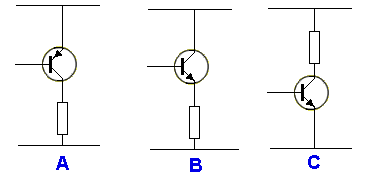TRANSISTOR TEST   Try this test before reading THE TRANSISTOR AMPLIFIER eBook and see how much you know: Question 1:  What is the voltage at point "X:"Choose from: 1. High Voltage 2. Mid-rail Voltage 3. Low Voltage   - Close window before scrolling Question 2:  What is the voltage at point "X:"Choose from: 1. High Voltage 2. Mid-rail Voltage 3. Low Voltage   Question 3:  What is the voltage at point "X:"Choose from: 1. High Voltage 2. Mid-rail Voltage 3. Low Voltage   Question 4:  What is the simplest way to turn this circuit into a DIGITAL CIRCUIT:Question 5:  Will the voltage at point X rise when the emitter-resistor is added:Question 6:  Identify the emitter-follower circuit:Question 7:  Identify the constant-current circuit:Question 8:  If you increase the value of the base-bias resistor, what will happen to the collector voltage?     Question 9:  What is the collector voltage:Question 10:  Draw a NAND gate using a transistor and diodes:       Now go to: THE TRANSISTOR AMPLIFIER and see how much you will learn.